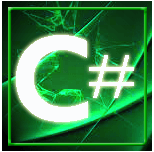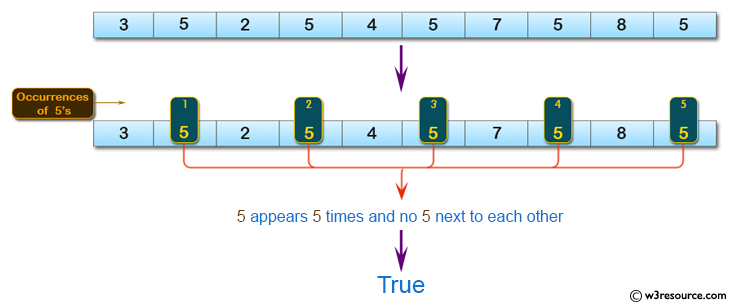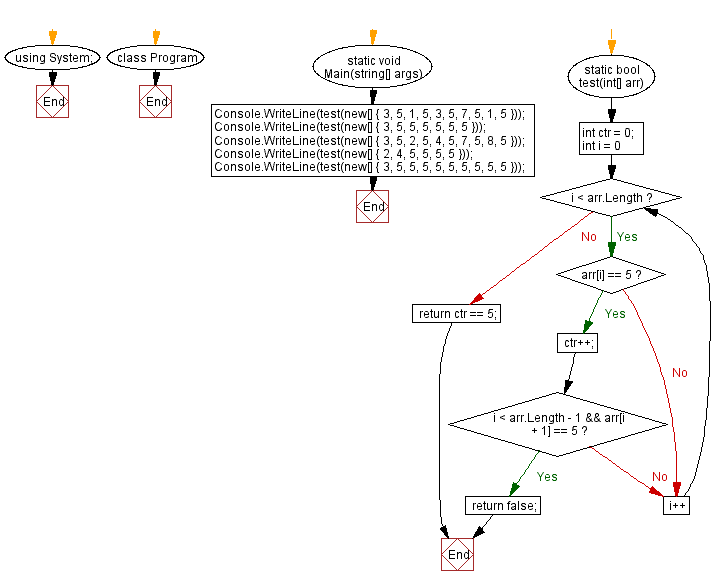﻿ C# Sharp exercises: Check a given array of integers and return true if the value 5 appears 5 times and there are no 5 next to each other - w3resource# C# Sharp Basic Algorithm Exercises: Check a given array of integers and return true if the value 5 appears 5 times and there are no 5 next to each other

## C# Sharp Basic Algorithm: Exercise-123 with Solution

Write a C# Sharp program to check a given array of integers and return true if the value 5 appears 5 times and there are no 5 next to each other.

Pictorial Presentation:Sample Solution:

C# Sharp Code:

``````using System;
namespace exercises
{
class Program
{
static void Main(string[] args)
{
Console.WriteLine(test(new[] { 3, 5, 1, 5, 3, 5, 7, 5, 1, 5 }));
Console.WriteLine(test(new[] { 3, 5, 5, 5, 5, 5, 5}));
Console.WriteLine(test(new[] { 3, 5, 2, 5, 4, 5, 7,  5, 8,  5}));
Console.WriteLine(test(new[] { 2, 4, 5, 5, 5, 5}));
}
static bool test(int[] numbers)
{
bool flag = false;
int five = 0;

for (int i = 0; i < numbers.Length; i++)
{
if (numbers[i] == 5 && !flag)
{
five++;
flag = true;
}
else
{
flag = false;
}
}

return five == 5;
}
}
}
```
```

Sample Output:

```True
False
True
False```

Flowchart:C# Sharp Code Editor:

Improve this sample solution and post your code through Disqus

What is the difficulty level of this exercise?

﻿

New Content: Composer: Dependency manager for PHP, R Programming